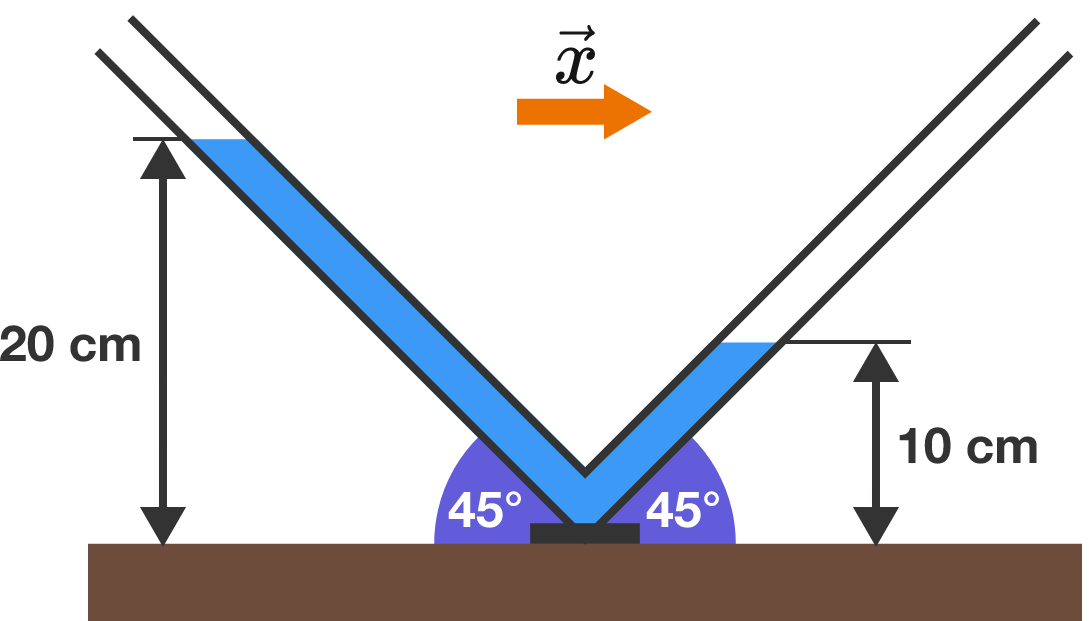# What's the acceleration?

An accelerometer is made using tubular sections, as shown below. The accelerometer is placed in a rocket that travels in the $\vec{x}$ direction, and the liquid levels are steady at $\SI{20}{\centi\meter}$ and $\SI{10}{\centi\meter}.$

Find the magnitude of the rocket’s acceleration (in $\text{m/s}^2$). Submit your answer to 3 decimal places.Details and Assumptions:

• The diameter of the tube is much smaller than the heights of the liquid in the two arms.
• $g = 10 \text{ m/s}^{2}.$
×